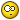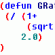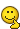# Sorting local variables? Is this possible?

## Recommended PostsHey everyone. Could use a little assistance here. Not sure if this is even possible, but I assume it should be. I'm still in the "i know enough LISP just to be dangerous, but slightly useful" stageI have a LISP routine that collects 5 variables from the user (r1,r2,r3,r4,r5).

```
(setq r1 (getint "\nEnter First Rev Line Number use ZERO for NONE: "))
(setq r2 (getint "\nEnter Second Rev Line Number use ZERO for NONE: "))
(if (= r2 0)(setq r3 0)(setq r3 (getint "\nEnter Third Rev Line Number use ZERO for NONE: ")))
(if (= r3 0)(setq r4 0)(setq r4 (getint "\nEnter Fourth Rev Line Number use ZERO for NONE: ")))
(if (= r4 0)(setq r5 0)(setq r5 (getint "\nEnter Fifth Rev Line Number use ZERO for NONE: ")))
```

What I would like to do with them, is sort them by their values, putting the highest value first, then sorting in reverse.

Can anyone offer any insight on this? Thank you in advance!

##### Share on other sitesLook into (vl-sort) It is a fairly simple function. -David

##### Share on other sitesThanks David. I looked up some of the syntax for the vl-sort.. seems like it should be simple enough!

##### Share on other sitesWell I tried getting this to work, but I cant seem to make it happen using my variables.

I have something like this:

```  (setq r1 (getint "\nEnter First Rev Line Number use ZERO for NONE: "))
(setq r2 (getint "\nEnter Second Rev Line Number use ZERO for NONE: "))
(setq r3 (getint "\nEnter Third Rev Line Number use ZERO for NONE: "))
(setq r4 (getint "\nEnter Fourth Rev Line Number use ZERO for NONE: "))
(setq r5 (getint "\nEnter Fifth Rev Line Number use ZERO for NONE: "))

(setq revs (vl-sort '(r1 r2 r3 r4 r5) '>))
(setq r1 (nth 0 revs)
r2 (nth 1 revs)
r3 (nth 2 revs)
r4 (nth 3 revs)
r5 (nth 4 revs))```

When I try running that, I get a "error: bad argument type for compare: R2 R1". This is all pretty much guesswork for me as far as writing the code, so anything anyone can do to help me out would be greatly appreciated!

Thanks!##### Share on other sitesBy using quote to define the list you prevent his items from being evaluated:

`(setq revs (vl-sort ([color=red]list[/color] r1 r2 r3 r4 r5) '>))`

Regards,

Mircea

##### Share on other sitesNice! Thanks!

Now all I need to do is figure out how to make it work if one of the values is zero...Getting a " ; error: bad argument type: fixnump: nil" now..

##### Share on other sitesPressing enter may be giving you a "nil" not a "0" there not the same

##### Share on other sitesNice! Thanks!

Now all I need to do is figure out how to make it work if one of the values is zero...Getting a " ; error: bad argument type: fixnump: nil" now..

Press Enter in Getint request will return nilIf you want return 0, you can re-write like this :

```(mapcar '(lambda(a b)(set a b))
'(r1 r2 r3 r4 r5)
(vl-sort
(mapcar '(lambda(a)(cond ((getint (strcat "\nEnter " a "Rev Line Number use ZERO for NONE: ")))(0)))
'("First" "Second" "Third" "Fourth" "Fifth")
)
'>
)
)
```

##### Share on other sitesA little more elementary usage would be:

```(initget 1)
(setq r1 (getint "\nEnter First Rev Line Number use ZERO for NONE: "))
(initget 1)
(setq r2 (getint "\nEnter Second Rev Line Number use ZERO for NONE: "))
```

(initget) uses bit flags

The basic values are:

1 = not nil

2 = not zero

4 = not negative

so (initget 7) : would force an input of a positive number > 0

-David

##### Share on other sitesThanks guys Ill have to give those a try.

I think I know the problem I'm running into.. In the code I tell it to sort 5 values, 2 or 3 of which may end up being 0. So after the list is sorted, the duplicates are removed, and if I try to pull the 4th and 5th values out of the list, they aren't there anymore.. So when the code tries to run:

`(setq r1 (nth 4 revs))`

.. that value basically doesn't exist anymore, thus returning the "nil" value. Am I understanding that correctly?

##### Share on other sitesAm I understanding that correctly?

Yes, this is true.

One simple alternative is to temporarily convert the revision indexes to strings and use ACAD_STRLSORT to sort them:

`(mapcar 'atoi (acad_strlsort (mapcar 'itoa '(5 0 3 0 1))))`

Regards,

Mircea

##### Share on other sitesAlso simple

(if (= r1 nil)(setq r1 0))

```(if (= r1 nil)(setq r1 0))
or preset
(setq vert 50)
(prompt "\nEnter Vertical scale:<")
(prin1 vert)
(prompt ">:")
(setq newvert (getint))
(if (= newvert nil)
(princ)
(setq vert newvert)
)```

## Join the conversation

You can post now and register later. If you have an account, sign in now to post with your account.
Note: Your post will require moderator approval before it will be visible.×   Pasted as rich text.   Paste as plain text instead

Only 75 emoji are allowed.